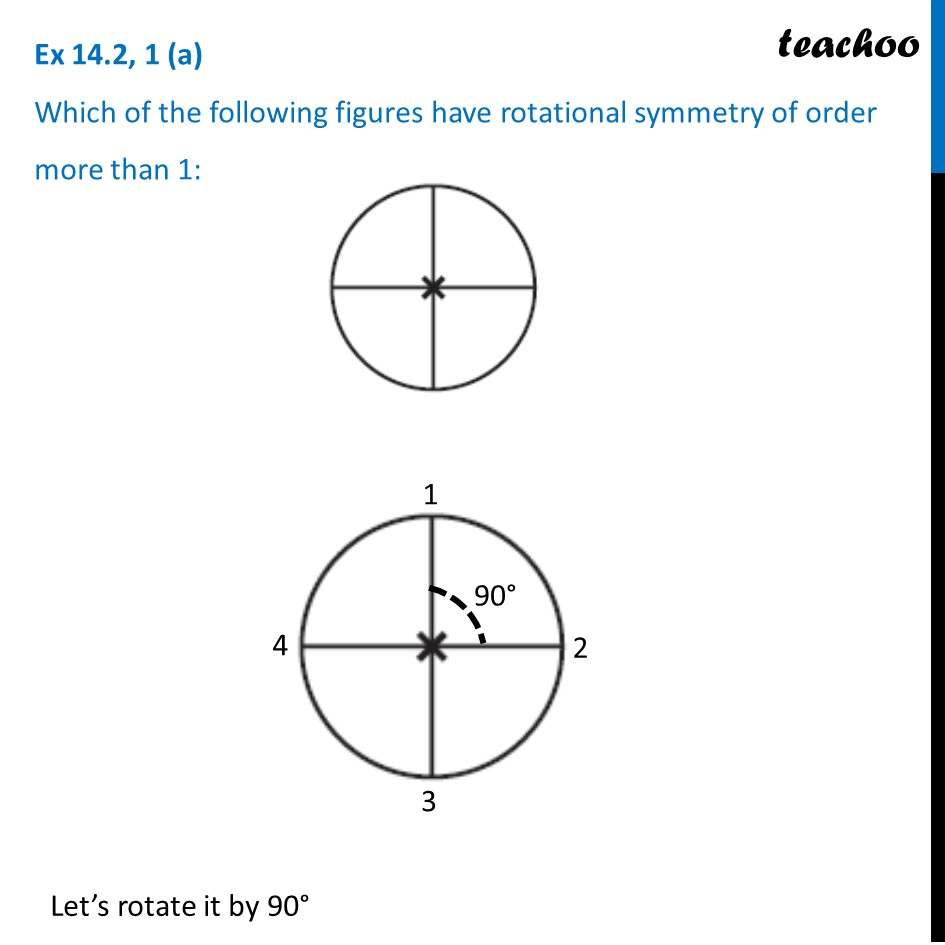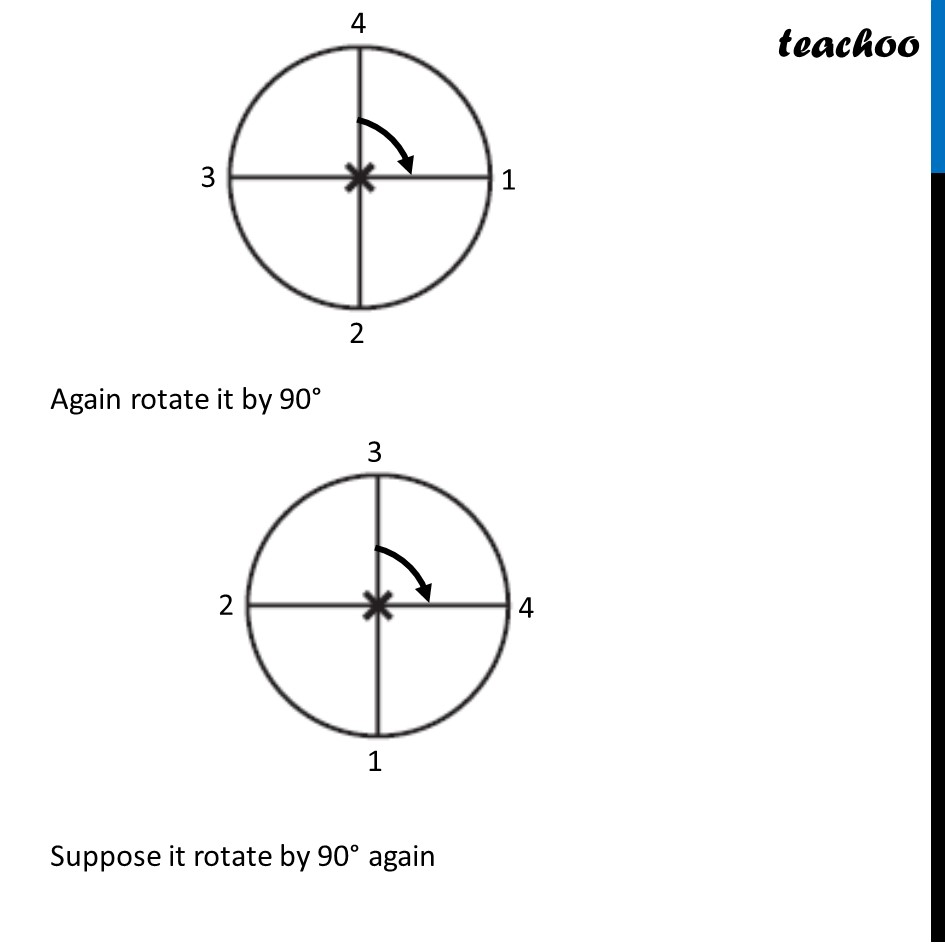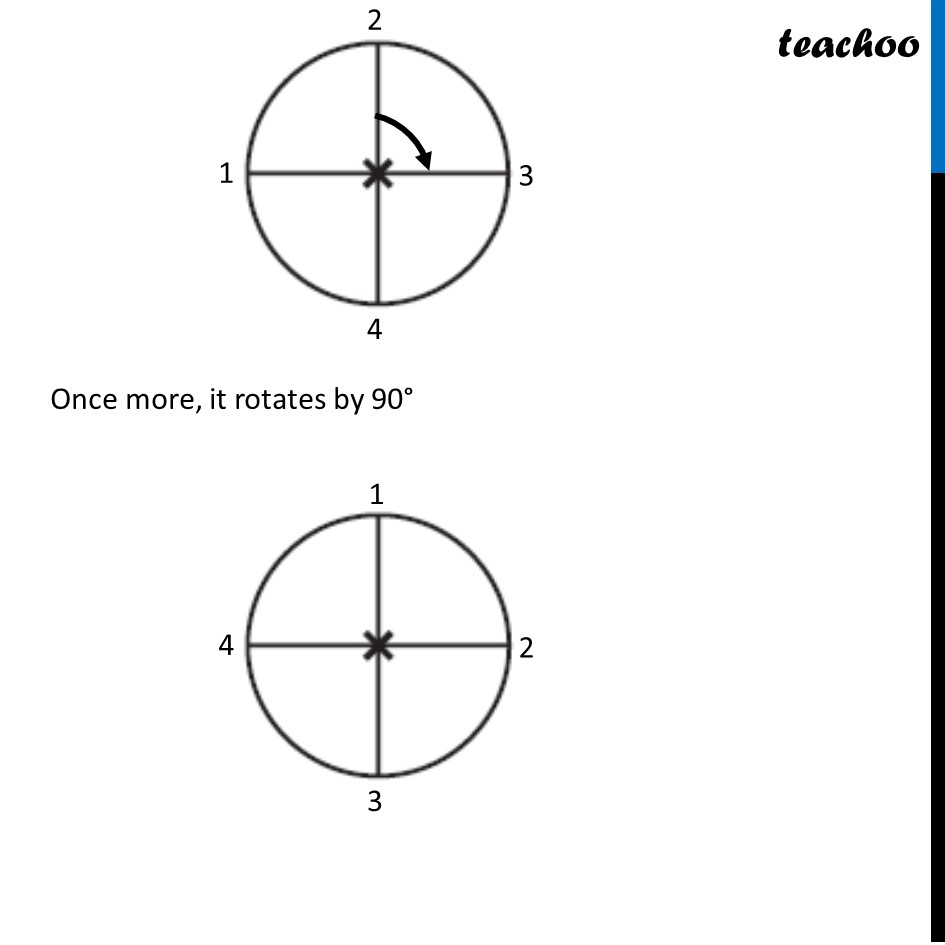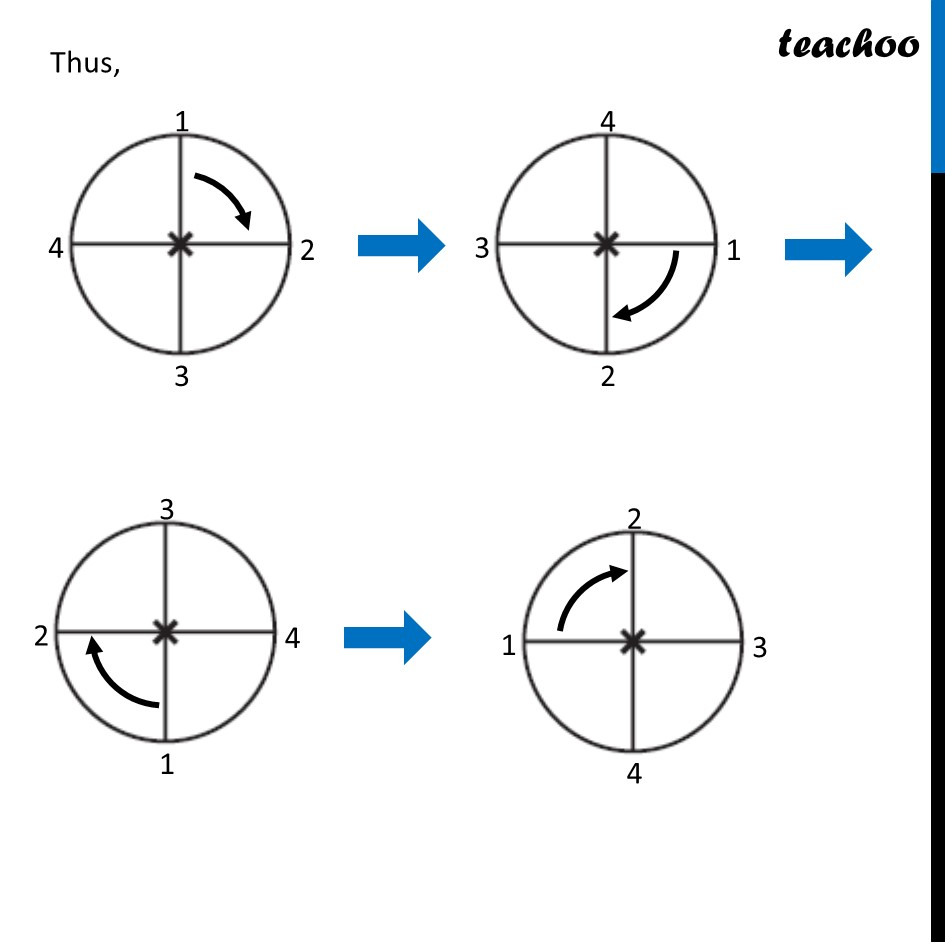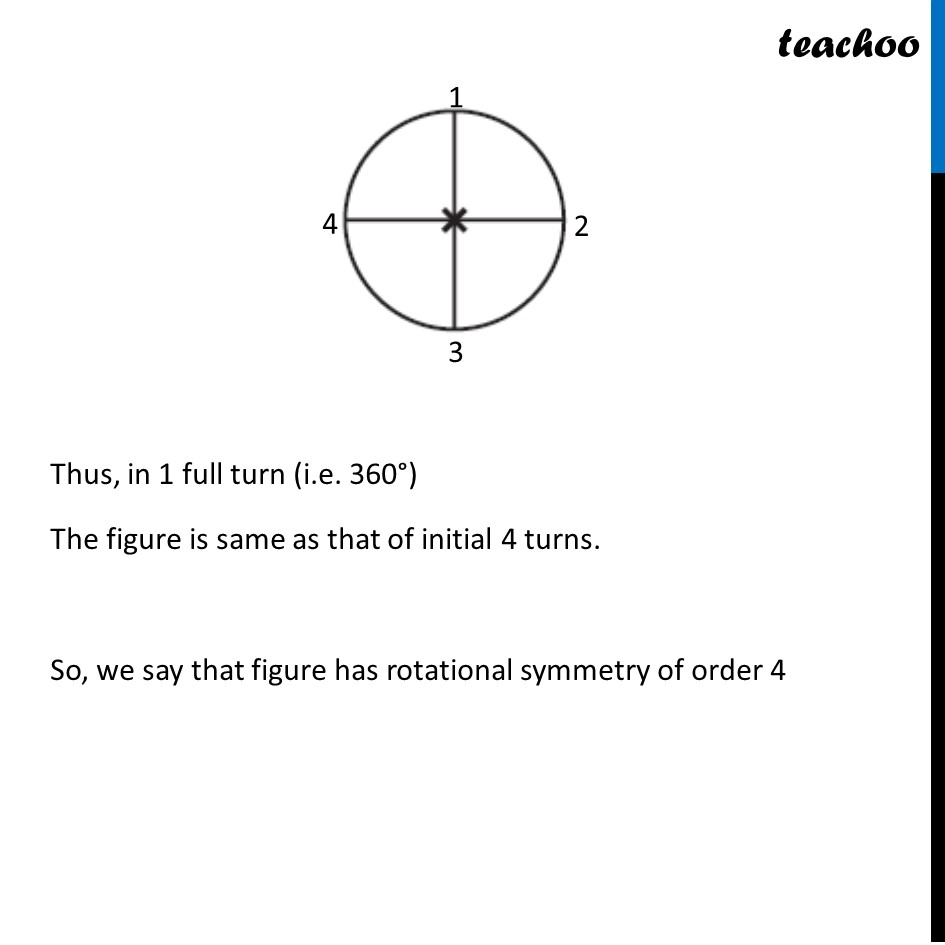1. Chapter 14 Class 7 Symmetry
2. Serial order wise
3. Ex 14.2

Transcript

Ex 14.2, 1 (a) Which of the following figures have rotational symmetry of order more than 1: Let’s rotate it by 90° Again rotate it by 90° Suppose it rotate by 90° again Once more, it rotates by 90° Thus, Thus, in 1 full turn (i.e. 360°) The figure is same as that of initial 4 turns. So, we say that figure has rotational symmetry of order 4

Ex 14.2

Chapter 14 Class 7 Symmetry
Serial order wise